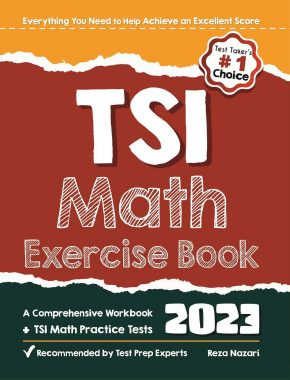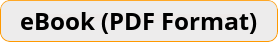\$13.99

# TSI Math Exercise Book A Comprehensive Workbook + TSI Math Practice TestsA Perfect TSIA 2.0 Math Practice Book!
TSI Math Exercise Book is designed to help you study all Math topics covered on the TSI test and to challenge you to get a high score on your actual TSI Math test. It not only has a ton of TSI Math practice questions, but it also has sample practice test and extensive explanations for each solution.Teacher's Choice
100% Guaranteed
Secure Checkout

TSI Math Exercise Book contains simple-to-follow directions, useful examples, as well as lots of practice math problems to help test takers master every concept, as well as brush up on their skill of solving problems and give them more self-confidence.

This complete and up-to-date exercise book covers every Math topic you’ll ever need to prepare for the TSIA 2.0 test. It has a plethora of math skill-building tasks and worksheets covering fundamental math, arithmetic, pre-algebra, algebra, geometry, basic statistics, probability, and a variety of other math topics. For each question, a detailed answer is provided.
Two full-length TSI Math exams with extensive explanations will help you enhance your Mathematics knowledge and prepare for the TSI Math examination.
TSI Math Exercise Book has unique features to help you prepare for the exam, including:
– Content 100% aligned with the 2023 TSI test
– Written by a top TSI Math tutor and test expert
– Complete coverage of all TSI Math concepts and topics which you will be tested
– Exercises on different TSI Math topics such as integers, percent, equations, polynomials, exponents, and radicals
– 2 full-length practice tests (featuring new question types) with detailed answers
– And much more!

After finishing this hands-on activity book, you will have gained confidence, a solid foundation, and enough experience to pass the TSI Math test.
Do not take the TSI without first mastering the Math problems in this Exercise book!

Ideal for self-study and classroom usage!